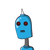# Eind the volume of the largest rightcircular cone that can bebe cut outof a cube whose edge is 18cm​

Eind the volume of the largest right
circular cone that can be
be cut out
of a cube whose edge is 18cm​

### 1 thought on “Eind the volume of the largest right<br />circular cone that can be<br />be cut out<br />of a cube whose edge is 18cm​”

1.486π cm³

Step-by-step explanation:

a = 18

So,

Radius of cone, r = 18/2 = 9 cm

Height of cone, h = 18 cm

V = 1/3 πr²h

V = 1/3 × π × 9² × 18

V = 486π cm³